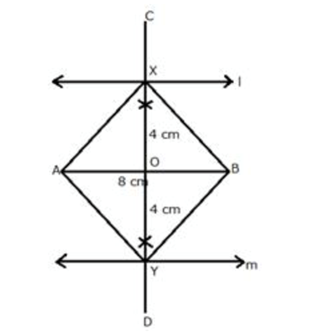## Ivy Online Glossary

Browse the glossary using this index

Special | A | B | C | D | E | F | G | H | I | J | K | L | M | N | O | P | Q | R | S | T | U | V | W | X | Y | Z | ALL

Page:  1  2  (Next)
ALL

### L

#### locus of a point

A straight-line AB is 8 cm long. Draw and describe the locus of a point which is: always 4 cm from the line AB

SolutionStep 1:

Draw a line segment AB of length 8 cm.

Step 2:

Draw two parallel lines l and m to AB at a distance of 4 cm.

Step 3:

Draw the perpendicular bisector of AB which intersects the parallel lines l and m, at X and Y respectively then, X and Y are the required points.

Step 4:

Join AX, AY, BX and BY.

The figure AXBY is a square as its diagonals are equal and intersect at 90o.

### Q

#### quadratic-equation-823

Page:  1  2  (Next)
ALL